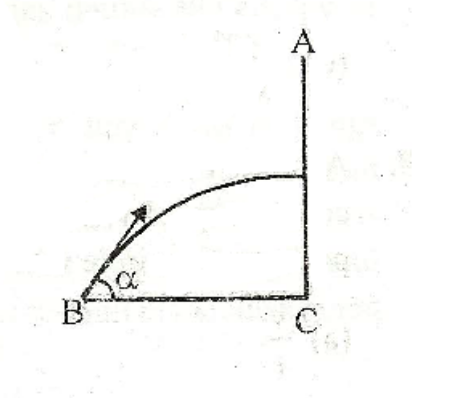# BullsEyeA body A is dropped froma a height $h$ above the ground. At the same time another body B at a distance $d$ from the projection of A from the ground is fired at an angle $\alpha$ to the horizontal. If the two collide at the maximum point of the trajectory of B the angle of the projection is given by

(A) $\alpha = \tan^{-1} \left( \frac dh \right)$

(B) $\alpha = \sin^{-1} \left( \frac hd \right)$

(C) $\alpha = \tan^{-1} \left( \frac hd \right)$

(D) $\alpha = \cos^{-1} \left( \frac hd \right)$

×